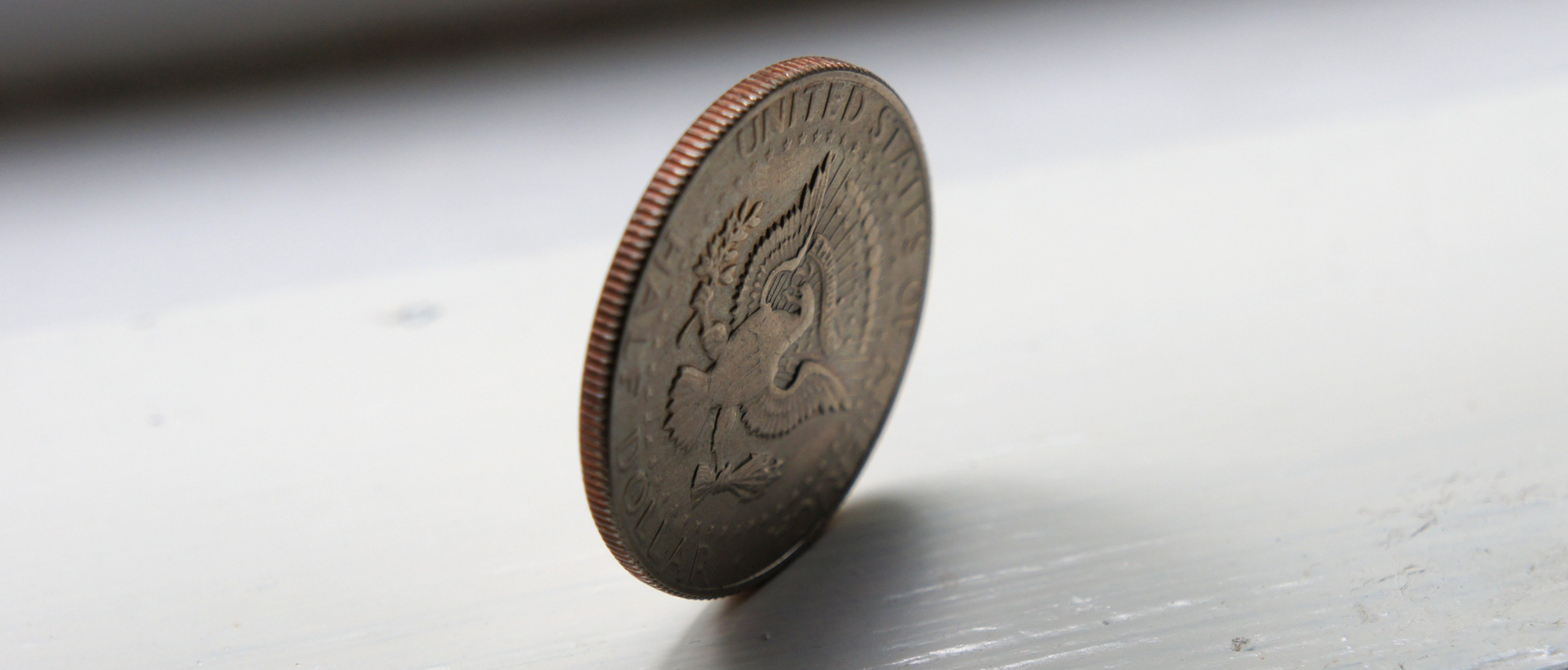An unexpected way to beat the odds in this classic gameHeads, tails or somewhere in-between. Image: Igor Motov, CC BY-NC-SA 2.0.

Recently I attended a quiz night at our local club. During the break the attendees played Heads and Tails. The rules are as follows:

 Heads and Tails   Two coins are thrown by the organiser. The three possible (but not equally likely) outcomes are 2 heads, 2 tails and a head and a tail. Players place their two hands on their heads, two hands on their bottom or one hand on their head and one hand on their bottom signifying that they are betting on the outcome being 2 heads, 2 tails or a head and a tail respectively. Those players guessing incorrectly are eliminated and the game continues until one person is left who is the winner.

The game proceeded in the usual way until there were five players left of which I was one. Before deciding where to place my hands I looked round to see that of the other four players one was opting for two heads, one was opting for two tails and two were opting for a head and a tail.

Since a head and a tail is the most likely outcome I opted for one hand on head and one hand on bottom. The quizmaster threw the coins and they landed two heads. The winner celebrated but the people in the room said he was lucky and that his choice was illogical.  The player, however, opting for two heads had a $\frac{1}{4}$ chance of winning and given that there were five players still in the pool it was a good choice – any other choice would have given a smaller probability than $\frac{1}{4}$. What should I have chosen?

$\mathbb{P}$( winning the game| Two heads chosen) = $\mathbb{P}$( Two heads)$\times\mathbb{P}$(winning from a pool of two players remaining)
If we assume the probability of winning from a pool of two players is $\frac{1}{2}$ then the probability of me winning is $\frac{1}{4}\times\frac{1}{2}=\frac{1}{8}$.
2. Choose two tails
$\mathbb{P}$(me winning the game| Two tails chosen) =$\mathbb{P}$(Two tails)$\times\mathbb{P}$(winning from a pool of two players remaining)
If we assume the probability of me winning from a pool of two players is $\frac{1}{2}$, then the probability of me winning is  $\frac{1}{4}\times\frac{1}{2}=\frac{1}{8}$.
3. Choose one head and one tail
$\mathbb{P}$(me winning the game| One head and one tail chosen) = \mathbb{P}( One head and one tail)$\times\mathbb{P}$(winning from a pool of $3$ players remaining)
If we assume that the probability of me winning from a pool of $3$ players is $\frac{1}{3}$, then the probability of me winning is $\frac{1}{2}\times\frac{1}{3}=\frac{1}{6}$.

Interestingly none of my options gives me a chance of winning that is as great as $\frac{1}{4}$. Whatever option I choose gives one or more of the other players a greater than $\frac{1}{4}$ chance of winning!

## General strategy

The question arises as to how one should play in general. If there are $N$ players in the pool (apart from oneself) and $n_1$ are opting for two heads, $n_2$ opting for two tails and $n$ opting for one head and one tail. i.e. there are $N + 1$ players and $n_1+ n_2+ n_3= N$

Consider the three options available to me:

$\mathbb{P}$(winning|Two heads chosen) =$\mathbb{P}$(Two heads)$\times\mathbb{P}$( winning from a pool of $n_1+ 1$)Assuming for the sake of argument that the probability of winning from a pool of $X$ players is $\frac{1}{X}$. We have that:

$\mathbb{P}$(winning| Two heads chosen) =$\frac{1}{4} \times \frac{1}{n_1+1}$

If $n_1 < \frac{N}{4} -1$ then the probability of winning is greater than $\frac{1}{N}$ and it is sensible to opt for two heads.

2. Choose two tails
A similar argument to that above shows that$\mathbb{P}$(winning| Two tails chosen) = $\frac{1}{4}\times\frac{1}{n_2+1}$.If $n_2 < \frac{N}{4}-1$ then the probability of winning is greater than $\frac{1}{N}$ and it is sensible to opt for two tails.
3. Choosing one head and one tail
$\mathbb{P}$(winning| One head and one tail chosen) =$\frac{1}{2}\times\frac{1}{n_3+1}$If $n_3 < \frac{N}{2}-1$ then the probability of winning is greater than $\frac{1}{N}$ and it is sensible to opt for one head and one tail.

Interestingly if $n_1=n_2=\frac{N}{4}$ and $n_3=\frac{N}{2}$ then there is no option that gives a probability of winning of less than $\frac{1}{N}$.

In this case the probabilities of winning are:

1. $\frac{1}{N+4}$
2. $\frac{1}{N+4}$
3. $\frac{1}{N+2}$

Hence the best option is to opt for one head and one tail.

In general if one is playing Heads and Tails one should, at the outset, consider the proportions of players opting for the three possibilities.

Imagine, for example, if there are 1000 people taking part and everyone else has opted for 1 head and 1 tail. If you opt for two heads you have a one in four chance of winning. Whereas if you opt for one head and one tail you have a 50% chance of not being eliminated and would still have to rely on the all those people being eliminated later on.

In general if less than 25% of the players are opting for two heads then opt for two heads, if less than 25% of the players are opting for two tails then opt for two tails and if less than 50% of the players are opting for one head and one tail then opt for one head and one tail.Mike is a former chief examiner for statistics, a lecturer in probability and a teaser writer for the Sunday Times.
+ More articles by Mike